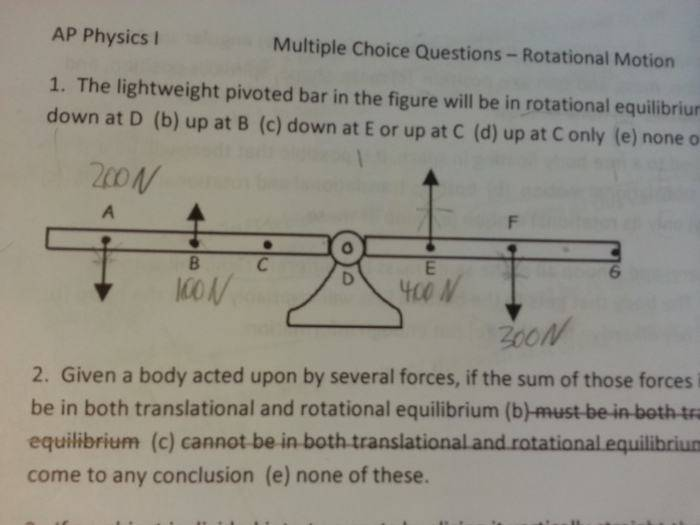# Rotational Motion - Conceptual

Soniteflash

## Homework Statement

The lightweight pivoted bar in the figure will be in rotational equilibrium when a 200N force acts(a) down at D
(b) up at B
(c) down at E or up at C
(d) up at C only
(e) none of these

## Homework Equations

Torque = Force x Distance
∑Torque= 0 for rotational equilibrium

## The Attempt at a Solution

I know that in order for the bar to be in rotational equilibrium, the sum of torques must equal zero. My problem is that the problem does not give me information about the distance the forces act. In my opinion the problem does not give me enough information to answer the problem correctly.

Therefore I chose e which I am not sure about either.

## Answers and Replies

Homework Helper
Gold Member
It looks to me as if you are supposed to assume the points are equally spaced, AB=BC=CD=DE=EF=FG
and to neglect the weight of the "light" bar.

•Soniteflash